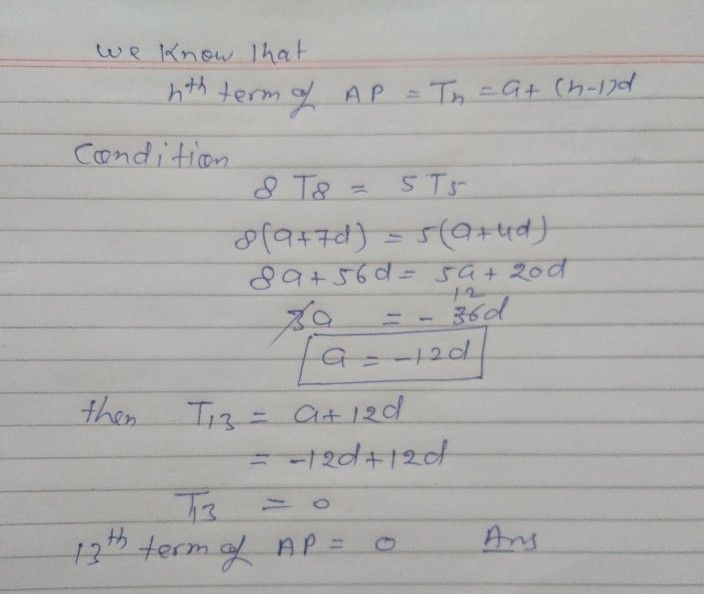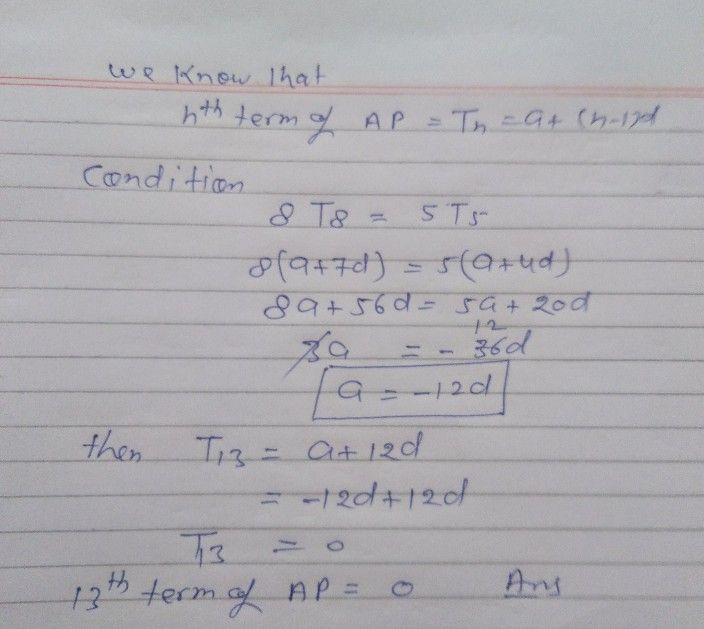Symbol
ProblemIf eight times $8^{1r}$ term of an $A$ AP is equal to five times $1$ its $5^{1}$ 5th term, then $1tS$ $13^{t}$ term is
10th-13th grade
Other
SolutionQanda teacher - Lokesh